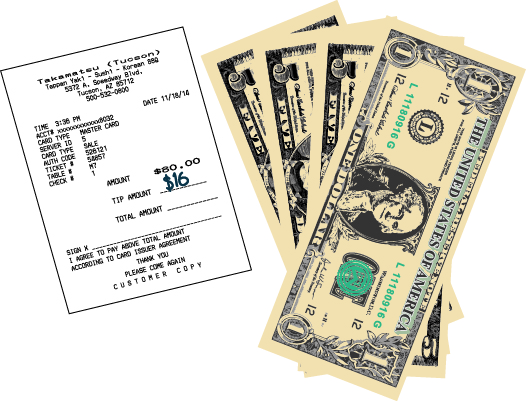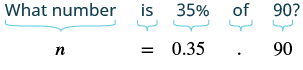Translating and Solving Basic Percent Equations

Learning Outcomes

• Solve percent equations for percent, amount, and base

We will solve percent equations by using the methods we used to solve equations with fractions or decimals. In the past, you may have solved percent problems by setting them up as proportions. That was the best method available when you did not have the tools of algebra. Now as a prealgebra student, you can translate word sentences into algebraic equations, and then solve the equations.

We’ll look at a common application of percent—tips to a server at a restaurant—to see how to set up a basic percent application.

When Aolani and her friends ate dinner at a restaurant, the bill came to $\text{\80}$. They wanted to leave a $\text{20%}$ tip. What amount would the tip be?

To solve this, we want to find what amount is $\text{20%}$ of $\text{\80}$. The $\text{\80}$ is called the base. The amount of the tip would be $0.20\left(80\right)$, or $\text{\16}$ See the image below. To find the amount of the tip, we multiplied the percent by the base.

A $\text{20%}$ tip for an $\text{\80}$ restaurant bill comes out to $\text{\16}$.Solve for Amount

In the next examples, we will find the amount. We must be sure to change the given percent to a decimal when we translate the words into an equation.

example

What number is $\text{35%}$ of $90?$

Solution

 Translate into algebra. Let $n=$ the number.Multiply. $n=31.5$ $31.5$ is $35\text{%}$ of $90$

example

$\text{125%}$ of $28$ is what number?

try it

In the next video we show another example of finding the base given a percent and the amount.

Solve for the Base

In the next examples, we are asked to find the base.

example

Translate and solve: $36$ is $\text{75%}$ of what number?

example

$\text{6.5%}$ of what number is $\text{\1.17}$?

try it

In the following video we show another example of how to find the base or whole given percent and amount.

Solve for the Percent

In the next examples, we will solve for the percent.

example

What percent of $36$ is $9?$

example

$144$ is what percent of $96?$

try it

In the next video we show another example of how to find the percent given amount and the base.